##### Basic Math & Pre-Algebra All-in-One For Dummies (+ Chapter Quizzes Online)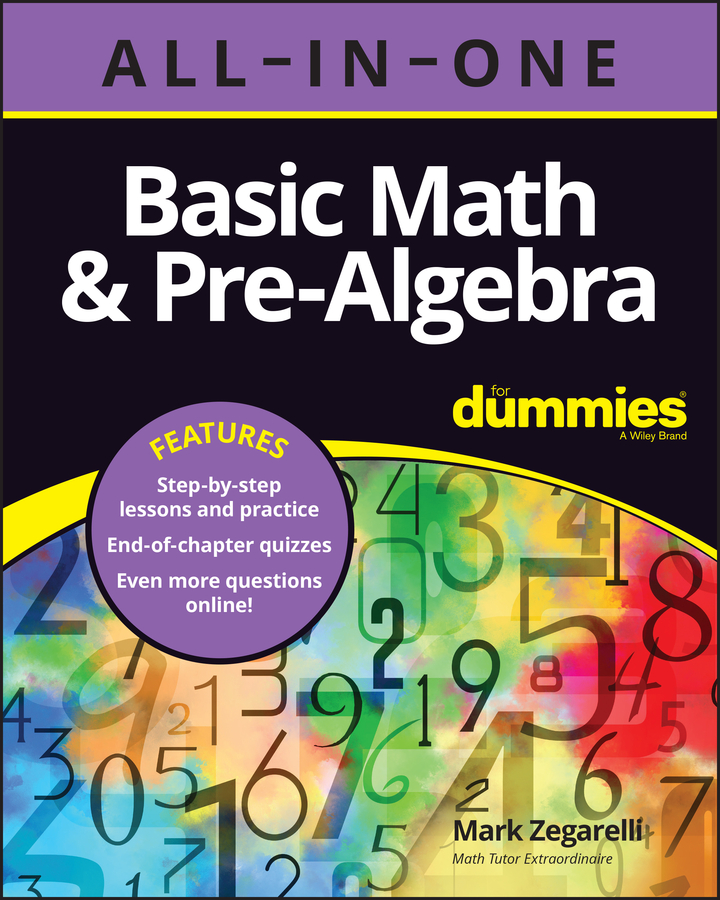To work with fractions, you need to know the different forms they take — reciprocal fractions, mixed numbers, proper and improper fractions — as well as the parts they’re made up of — denominators, numerators, and fraction bars.

Fractions are useful when you deal with numbers that do not represent whole units. For example, when you cut a cake into two equal pieces, each piece is half of the cake. As a fraction, you write that as 1/2. In the following figure, the shaded piece is half of the cake.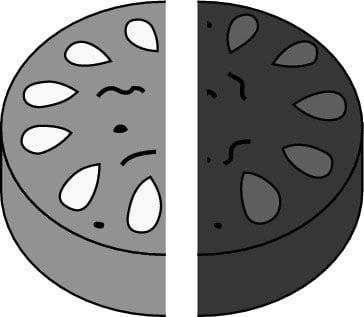Every fraction is made up of two numbers separated by a line, or a fraction bar. The line can be either diagonal or horizontal — so you can write this fraction in either of the following two ways: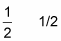The number above the line is called the numerator. The numerator tells you how many pieces you have. In this case, you have one dark-shaded piece of cake, so the numerator is 1.

The number below the line is called the denominator. The denominator tells you how many equal pieces the whole cake has been cut up into. In this case, the denominator is 2.

Similarly, when you cut a cake into three equal slices, each piece is a third of the cake, as the following figure shows.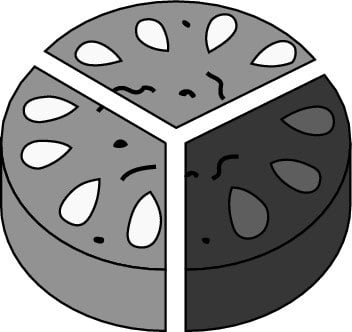This time, the shaded piece is one-third (1/3) of the cake. Again, the numerator tells you how many pieces you have, and the denominator tells you how many equal pieces the whole cake has been cut up into.

The following figure shows a few more examples of ways to represent parts of the whole with fractions.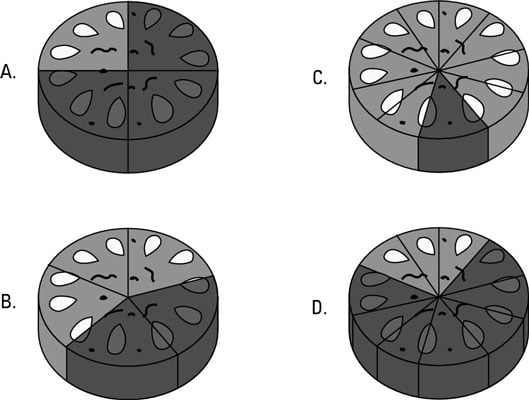In the above figure:

• A. = 3/4

• B. = 2/5

• C. = 1/10

• D. = 7/10

In each case, the numerator tells you how many pieces are shaded, and the denominator tells how many pieces there are altogether.

The fraction bar can also mean a division sign. In other words, 3/4 signifies 3 ÷ 4. If you take three cakes and divide them among four people, each person gets 3/4 of a cake.

## Reciprocal fractions

When you flip a fraction over, you get its reciprocal. For example, the following numbers are reciprocals: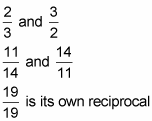## Ones and zeros in fractions

When the denominator (bottom number) of a fraction is 1, the fraction is equal to the numerator by itself. Or conversely, you can turn any whole number into a fraction by drawing a line and placing the number 1 under it. For example,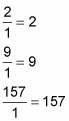When the numerator and denominator match, the fraction equals 1. That’s because if you cut a cake into eight pieces and you keep all eight of them, you have the entire cake. Here are some fractions that equal 1: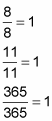When the numerator of a fraction is 0, the fraction is equal to 0. For example,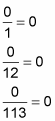The denominator of a fraction can never be 0. Fractions with 0 in the denominator are undefined — that is, they have no mathematical meaning.

## Mixed numbers

A mixed number is a combination of a whole number and a proper fraction added together. Here are some examples: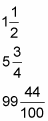A mixed number is always equal to the whole number plus the fraction attached to it.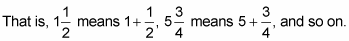## Proper fractions versus improper fractions

When the numerator and the denominator are equal, the fraction equals 1: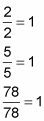When the numerator (top number) is less than the denominator (bottom number), the fraction is less than 1: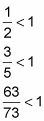Fractions like these are called proper fractions. Positive proper fractions are always between 0 and 1. However, when the numerator is greater than the denominator, the fraction is greater than 1. Take a look: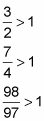Any fraction that’s greater than 1 is called an improper fraction. It’s customary to convert an improper fraction to a mixed number, especially when it’s the final answer to a problem.

An improper fraction is always top heavy, as if it’s unstable and wants to fall over. To stabilize it, convert it to a mixed number. On the other hand, proper fractions are always stable.